# Selina solutions for Concise Mathematics Class 7 ICSE chapter 14 - Lines and Angles (Including Construction of angles) [Latest edition]

#### Chapters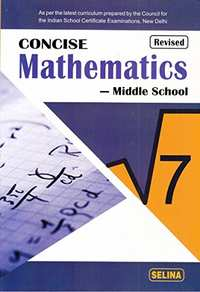## Chapter 14: Lines and Angles (Including Construction of angles)

Exercise 14 (A)Exercise 14 (B)Exercise 14 (C)
Exercise 14 (A)

### Selina solutions for Concise Mathematics Class 7 ICSE Chapter 14 Lines and Angles (Including Construction of angles) Exercise 14 (A)

Exercise 14 (A) | Q 1.01

State, true or false:

A line segment 4 cm long can have only 2000 points in it.

• True

• False

Exercise 14 (A) | Q 1.02

State, true or false:

A ray has one endpoint and a line segment has two end-points.

• True

• False

Exercise 14 (A) | Q 1.03

State, true or false:

A line segment is the shortest distance between any two given points.

• True

• False

Exercise 14 (A) | Q 1.04

State, true or false:

An infinite number of straight lines can be drawn through a given point.

• True

• False

Exercise 14 (A) | Q 1.05

Write the number of endpoints in
(a) a line segment AB
(b) a ray AB
(c) a line AB

Exercise 14 (A) | Q 1.06

out of $\overleftrightarrow{AB},\overrightarrow{AB},\overleftarrow{AB}$ and overline(AB) which one has a fixed length?

Exercise 14 (A) | Q 1.07

How many rays can be drawn through a fixed point O?

Exercise 14 (A) | Q 1.08

How many lines can be drawn through three
(a) collinear points?
(b) non-collinear points?

Exercise 14 (A) | Q 1.09

State, true or false:

Is 40° the complement of 60°?

• True

• False

Exercise 14 (A) | Q 1.1

State, true or false:

Is 45° the supplement of 45°?

• True

• False

Exercise 14 (A) | Q 2.1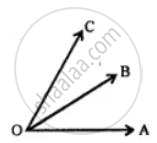Exercise 14 (A) | Q 2.2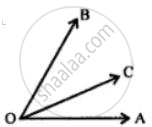Exercise 14 (A) | Q 2.3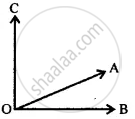Exercise 14 (A) | Q 2.4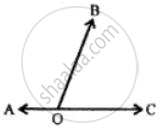Exercise 14 (A) | Q 3

In the given figure, BAC is a straight line.
Find:
(i) x
(ii) ∠AOB
(iii) ∠BOC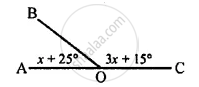Exercise 14 (A) | Q 4

Find y in the given figure.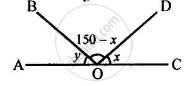Exercise 14 (A) | Q 5

In the given figure, find ∠PQR.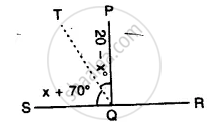Exercise 14 (A) | Q 6

In the given figure. p° = q° = r°, find each.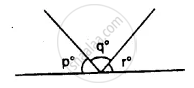Exercise 14 (A) | Q 7

In the given figure, if x = 2y, find x and y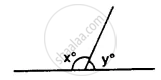Exercise 14 (A) | Q 8

In the adjoining figure, if b° = a° + c°, find b.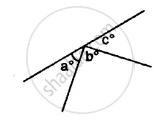Exercise 14 (A) | Q 9

In the given figure, AB is perpendicular to BC at B.
Find:
(i) the value of x.
(ii) the complement of angle x.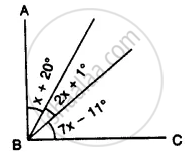Exercise 14 (A) | Q 10.1

Write the complement of 25°

Exercise 14 (A) | Q 10.2

Write the complement of 90°

Exercise 14 (A) | Q 10.3

Write the complement of a°

Exercise 14 (A) | Q 10.4

Write the complement of (x + 5)°

Exercise 14 (A) | Q 10.5

Write the complement of (30 − a)°

Exercise 14 (A) | Q 10.6

Write the complement of 1/2 of a right angle

Exercise 14 (A) | Q 10.7

Write the complement of 1/3 of 180°

Exercise 14 (A) | Q 10.8

Write the complement of 21° 17'

Exercise 14 (A) | Q 11.1

Write the supplement of 100°

Exercise 14 (A) | Q 11.2

Write the supplement of 0°

Exercise 14 (A) | Q 11.3

Write the supplement of x°

Exercise 14 (A) | Q 11.4

Write the supplement of (x + 35)°

Exercise 14 (A) | Q 11.5

Write the supplement of (90 + a + b)°

Exercise 14 (A) | Q 11.6

Write the supplement of (110 – x – 2y)°

Exercise 14 (A) | Q 11.7

Write the supplement of 1/5 of a right angle

Exercise 14 (A) | Q 11.8

Write the supplement of 80° 49′ 25″

Exercise 14 (A) | Q 12.1

Are the following pairs of angles complementary?
10° and 80°

Exercise 14 (A) | Q 12.2

Are the following pairs of angles complementary?
37° 28′ and 52° 33′

Exercise 14 (A) | Q 12.3

Are the following pairs of angles complementary?
(x + 16)° and (74 − x)°

Exercise 14 (A) | Q 12.4

Are the following pairs of angles complementary?
54° and 2/5 of a right angle.

Exercise 14 (A) | Q 13.1

Are the following pairs of angles supplementary?
139° and 39°.

Exercise 14 (A) | Q 13.2

Are the following pairs of angles supplementary?
26° 59′ and 153° 1′.

Exercise 14 (A) | Q 13.3

Are the following pairs of angles supplementary?
3/10 of a right angle and 4/15 of two right angles.

Exercise 14 (A) | Q 13.4

Are the following pairs of angles supplementary?
2x° + 65° and 115°− 2x°

Exercise 14 (A) | Q 14

If 3x + 18° and 2x + 25° are supplementary, find the value of x.

Exercise 14 (A) | Q 15

If two complementary angles are in the ratio 1 : 5, find them.

Exercise 14 (A) | Q 16

If two supplementary angles are in the ratio 2 : 7, find them.

Exercise 14 (A) | Q 17

Three angles which add up to 180° are in the ratio 2 : 3 : 7. Find them.

Exercise 14 (A) | Q 18

20% of an angle is the supplement of 60°. Find the angle.

Exercise 14 (A) | Q 19

10% of x° is the complement of 40% of 2x°. Find x

Exercise 14 (A) | Q 20

Use the adjacent figure, to find angle x and its supplement.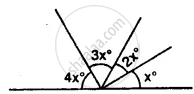Exercise 14 (A) | Q 21.1

Find k of the given figure.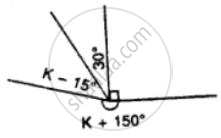Exercise 14 (A) | Q 21.2

Find k of the given figure.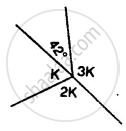Exercise 14 (A) | Q 22

In the given figure, lines PQ, MN, and RS intersect at O. If x : y = 1 : 2 and z = 90°, find ∠ROM and ∠POR.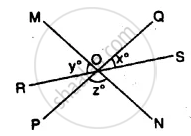Exercise 14 (A) | Q 23

In the given figure, find ∠AOB and ∠BOC.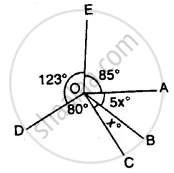Exercise 14 (A) | Q 24

Find each angle shown in the diagram.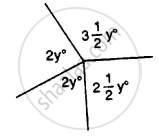Exercise 14 (A) | Q 25

AB, CD and EF are three lines intersecting at the same point.
(i) Find x, if y = 45° and z = 90°.
(ii) Find a, if x = 3a, y = 5x and r = 6x.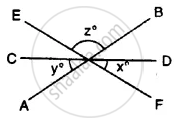Exercise 14 (B)

### Selina solutions for Concise Mathematics Class 7 ICSE Chapter 14 Lines and Angles (Including Construction of angles) Exercise 14 (B)

Exercise 14 (B) | Q 1.1

Identify the given pair of angles as ∠3 and ∠6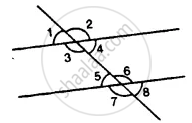• corresponding angles

• interior alternate angles

• exterior alternate angles

• vertically opposite angles

• allied angles

Exercise 14 (B) | Q 1.2

Identify the given pair of angles as ∠2 and ∠4• corresponding angles

• interior alternate angles

• exterior alternate angles

• vertically opposite angles

• allied angles

Exercise 14 (B) | Q 1.3

Identify the given pair of angles as ∠3 and ∠7• corresponding angles

• interior alternate angles

• exterior alternate angles

• vertically opposite angles

• allied angles

Exercise 14 (B) | Q 1.4

Identify the given pair of angles as ∠2 and ∠7• corresponding angles

• interior alternate angles

• exterior alternate angles

• vertically opposite angles

• allied angles

Exercise 14 (B) | Q 1.5

Identify the given pair of angles as ∠4 and ∠6• corresponding angles

• interior alternate angles

• exterior alternate angles

• vertically opposite angles

• allied or co-interior angles

Exercise 14 (B) | Q 1.6

Identify the given pair of angles as ∠1 and ∠8• corresponding angles

• interior alternate angles

• exterior alternate angles

• vertically opposite angles

• allied angles

Exercise 14 (B) | Q 1.7

Identify the given pair of angles as ∠1 and ∠5• corresponding angles

• interior alternate angles

• exterior alternate angles

• vertically opposite angles

• allied angles

Exercise 14 (B) | Q 1.8

Identify the given pair of angles as ∠1 and ∠4• corresponding angles

• interior alternate angles

• exterior alternate angles

• vertically opposite angles

• allied angles

Exercise 14 (B) | Q 1.9

Identify the given pair of angles as ∠5 and ∠7• corresponding angles

• interior alternate angles

• exterior alternate angles

• vertically opposite angles

• allied angles

Exercise 14 (B) | Q 2.01

Identify the given pair of angles as ∠1 and ∠4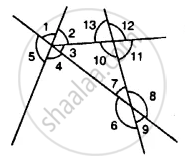• corresponding angles

• interior alternate angles

• exterior alternate angles

• vertically opposite angles

• allied angles

Exercise 14 (B) | Q 2.02

Identify the given pair of angles as ∠4 and ∠7• corresponding angles

• interior alternate angles

• exterior alternate angles

• vertically opposite angles

• allied angles

Exercise 14 (B) | Q 2.03

Identify the given pair of angles as ∠10 and ∠12• corresponding angles

• interior alternate angles

• exterior alternate angles

• vertically opposite angles

• allied angles

Exercise 14 (B) | Q 2.04

Identify the given pair of angles as ∠7 and ∠13• corresponding angles

• interior alternate angles

• exterior alternate angles

• vertically opposite angles

• allied angles

Exercise 14 (B) | Q 2.05

Identify the given pair of angles as ∠6 and ∠8• corresponding angles

• interior alternate angles

• exterior alternate angles

• vertically opposite angles

• allied angles

Exercise 14 (B) | Q 2.06

Identify the given pair of angles as ∠11 and ∠8• corresponding angles

• interior alternate angles

• exterior alternate angles

• vertically opposite angles

• allied or co-interior angles

Exercise 14 (B) | Q 2.07

Identify the given pair of angles as ∠7 and ∠9• corresponding angles

• interior alternate angles

• exterior alternate angles

• vertically opposite angles

• allied angles

Exercise 14 (B) | Q 2.08

Identify the given pair of angles as ∠4 and ∠5• corresponding angles

• interior alternate angles

• exterior alternate angles

• vertically opposite angles

• allied angles

Exercise 14 (B) | Q 2.09

Identify the given pair of angles as ∠4 and ∠6• corresponding angles

• interior alternate angles

• exterior alternate angles

• vertically opposite angles

• allied or co-interior angles

Exercise 14 (B) | Q 2.1

Identify the given pair of angles as ∠6 and ∠7• corresponding angles

• interior alternate angles

• exterior alternate angles

• vertically opposite angles

• allied angles

Exercise 14 (B) | Q 2.11

Identify the given pair of angles as ∠2 and ∠13• corresponding angles

• interior alternate angles

• exterior alternate angles

• vertically opposite angles

• allied or co-interior angles

Exercise 14 (B) | Q 3.1

In the given figure, the arrows indicate parallel lines. State which angles are equal. Give a reason.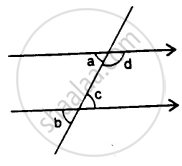Exercise 14 (B) | Q 3.2

In the given figure, the arrows indicate parallel lines. State which angles are equal. Give a reason.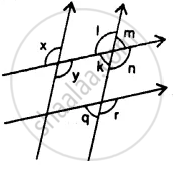Exercise 14 (B) | Q 4

In the given figure, find the measure of the unknown angles: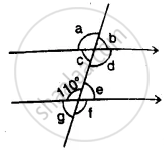Exercise 14 (B) | Q 5.1

Which pair of the dotted line, segments, in the following figure, are parallel. Give reason: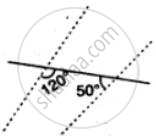Exercise 14 (B) | Q 5.2

Which pair of the dotted line, segments, in the following figure, are parallel. Give reason: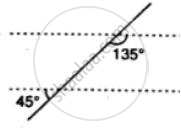Exercise 14 (B) | Q 5.3

Which pair of the dotted line, segments, in the following figure, are parallel. Give reason: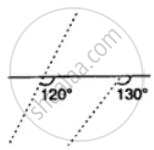Exercise 14 (B) | Q 5.4

Which pair of the dotted line, segments, in the following figure, are parallel. Give reason: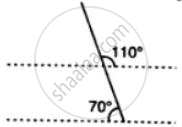Exercise 14 (B) | Q 5.5

Which pair of the dotted line, segments, in the following figure, are parallel. Give reason: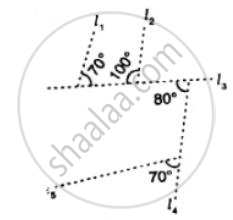Exercise 14 (B) | Q 5.6

Which pair of the dotted line, segments, in the following figure, are parallel. Give reason: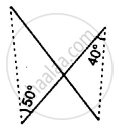Exercise 14 (B) | Q 6.01

In the given figure, the directed lines are parallel to each other. Find the unknown angles.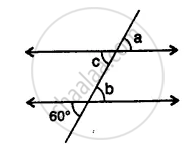Exercise 14 (B) | Q 6.02

In the given figure, the directed lines are parallel to each other. Find the unknown angles.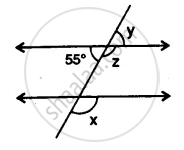Exercise 14 (B) | Q 6.03

In the given figure, the directed lines are parallel to each other. Find the unknown angles.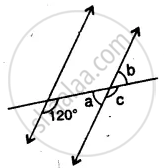Exercise 14 (B) | Q 6.04

In the given figure, the directed lines are parallel to each other. Find the unknown angles.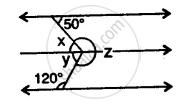Exercise 14 (B) | Q 6.05

In the given figure, the directed lines are parallel to each other. Find the unknown angles.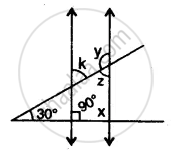Exercise 14 (B) | Q 6.06

In the given figure, the directed lines are parallel to each other. Find the unknown angles.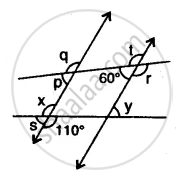Exercise 14 (B) | Q 6.07

In the given figure, the directed lines are parallel to each other. Find the unknown angles.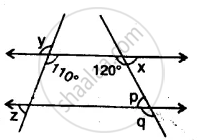Exercise 14 (B) | Q 6.08

In the given figure, the directed lines are parallel to each other. Find the unknown angles.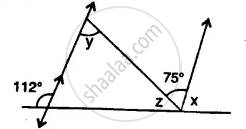Exercise 14 (B) | Q 6.09

In the given figure, the directed lines are parallel to each other. Find the unknown angles.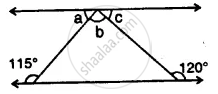Exercise 14 (B) | Q 6.1

In the given figure, the directed lines are parallel to each other. Find the unknown angles.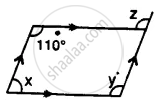Exercise 14 (B) | Q 6.11

In the given figure, the directed lines are parallel to each other. Find the unknown angles.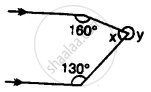Exercise 14 (B) | Q 6.12

In the given figure, the directed lines are parallel to each other. Find the unknown angles.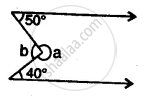Exercise 14 (B) | Q 7.1

Find x, y and p is the given figure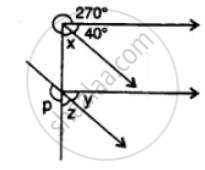Exercise 14 (B) | Q 7.2

Find x, y and p is the given figure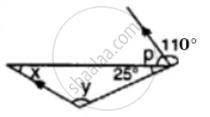Exercise 14 (B) | Q 8.1

Find x in the following case: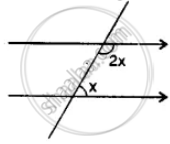Exercise 14 (B) | Q 8.2

Find x in the following case: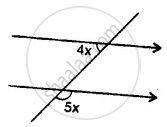Exercise 14 (B) | Q 8.3

Find x in the following case: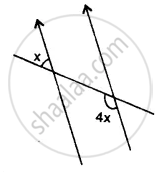Exercise 14 (B) | Q 8.4

Find x in the following case: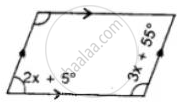Exercise 14 (B) | Q 8.5

Find x in the following case: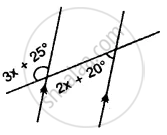Exercise 14 (B) | Q 8.6

Find x in the following case: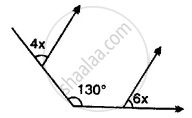Exercise 14 (C)

### Selina solutions for Concise Mathematics Class 7 ICSE Chapter 14 Lines and Angles (Including Construction of angles) Exercise 14 (C)

Exercise 14 (C) | Q 1.1

Using ruler and compasses, construct the following angle:
30°

Exercise 14 (C) | Q 1.2

Using ruler and compasses, construct the following angle:
15°

Exercise 14 (C) | Q 1.3

Using ruler and compasses, construct the following angle:
75°

Exercise 14 (C) | Q 1.4

Using ruler and compasses, construct the following angle:
180°

Exercise 14 (C) | Q 1.5

Using ruler and compasses, construct the following angle:
165°

Exercise 14 (C) | Q 1.6

Using ruler and compasses, construct the following angle:
22.5°

Exercise 14 (C) | Q 1.7

Using ruler and compasses, construct the following angle:
37.5°

Exercise 14 (C) | Q 1.8

Using ruler and compasses, construct the following angle:
67.5°

Exercise 14 (C) | Q 2

Draw ∠ABC = 120°. Bisect the angle using ruler and compasses only. Measure each 1 angle so obtained and check whether the angles obtained on bisecting ∠ABC are equal or not.

Exercise 14 (C) | Q 3

Draw a line segment PQ = 6 cm. Mark a point A in PQ so that AP = 2 cm. At point A, construct angle QAR = 60°.

Exercise 14 (C) | Q 4

Draw a line segment AB = 8 cm. Mark a point P in AB so that AP = 5 cm. At P, construct angle APQ = 30°.

Exercise 14 (C) | Q 5

Construct an angle of 75° and then bisect it.

Exercise 14 (C) | Q 6

Draw a line segment of length 6.4 cm. Draw its perpendicular bisector.

Exercise 14 (C) | Q 7

Draw a line segment AB = 5.8 cm. Mark a point P in AB such that PB = 3.6 cm. At P, draw a perpendicular to AB.

Exercise 14 (C) | Q 8.1

In the case, given below, draw a line through point P and parallel to AB: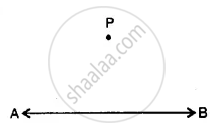Exercise 14 (C) | Q 8.2

In the case, given below, draw a line through point P and parallel to AB: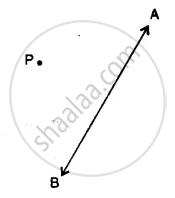Exercise 14 (C) | Q 8.3

In the case, given below, draw a line through point P and parallel to AB: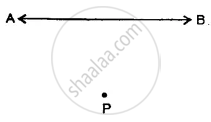## Chapter 14: Lines and Angles (Including Construction of angles)

Exercise 14 (A)Exercise 14 (B)Exercise 14 (C)## Selina solutions for Concise Mathematics Class 7 ICSE chapter 14 - Lines and Angles (Including Construction of angles)

Selina solutions for Concise Mathematics Class 7 ICSE chapter 14 (Lines and Angles (Including Construction of angles)) include all questions with solution and detail explanation. This will clear students doubts about any question and improve application skills while preparing for board exams. The detailed, step-by-step solutions will help you understand the concepts better and clear your confusions, if any. Shaalaa.com has the CISCE Concise Mathematics Class 7 ICSE solutions in a manner that help students grasp basic concepts better and faster.

Further, we at Shaalaa.com provide such solutions so that students can prepare for written exams. Selina textbook solutions can be a core help for self-study and acts as a perfect self-help guidance for students.

Concepts covered in Concise Mathematics Class 7 ICSE chapter 14 Lines and Angles (Including Construction of angles) are Concept for Pairs of Angles, Concept for Properties of Parallel Lines with Transversal, Construction of a Line Parallel to a Given Line from a Point Outside It ., Introduction to Lines and Angles.

Using Selina Class 7 solutions Lines and Angles (Including Construction of angles) exercise by students are an easy way to prepare for the exams, as they involve solutions arranged chapter-wise also page wise. The questions involved in Selina Solutions are important questions that can be asked in the final exam. Maximum students of CISCE Class 7 prefer Selina Textbook Solutions to score more in exam.

Get the free view of chapter 14 Lines and Angles (Including Construction of angles) Class 7 extra questions for Concise Mathematics Class 7 ICSE and can use Shaalaa.com to keep it handy for your exam preparation